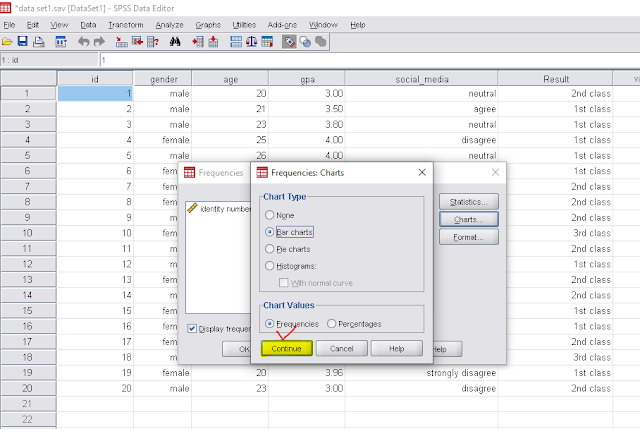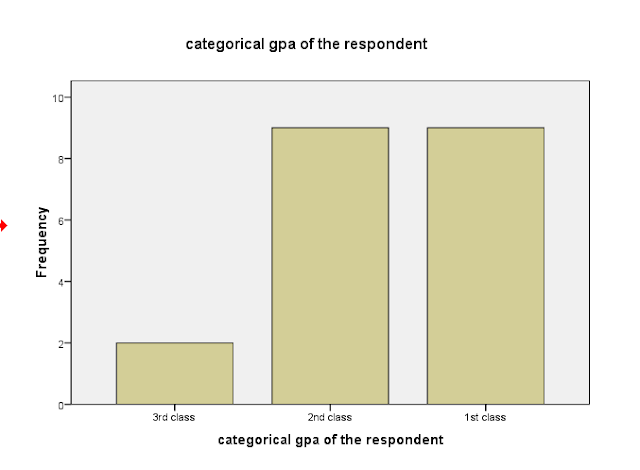# Univariate analysis| how to analyze data using spss (part-9)

What is univariate analysis?

Univariate analysis is the analysis of one variable such as descriptive statistics (mean, variance etc.).
We have the following data set with the several highlighted variable. Now we have to go to the following steps.Data set

Let we have five variables named gender, age, gpa, social_media and result. If we analyze each of the variable separately then its called univariate analysis. we can find the mean, variance and other descriptive statistics using univariate analysis. For univariate analysis first we have to go to--

1.Analyze →→Descriptive statistics→→ Frequencies

Now we have to select all the variable and taking them into the empty box named Variable(s).Frequencies

2.we have to click on StatisticsFrequencies

3. Select all the required area such as mean, median, standard deviation etc and click on Continue.Frequencies statistics

4. if we want the graph such as bar chart, pie chart then click on Charts and select the chart types and click on continue.Frequencies chart

5. click on Ok .Select OK

6. Now we can see the output window as following.FrequenciesBar chart

How to analyze data using SPSS (for beginners)-part 1
How to analyze data using SPSS (part-2), how to input data in SPSS
How to analyze data using SPSS (part-3), how to import data in SPSS from excel file
How to analyze data using SPSS (part-4), how to sort data in SPSS
How to analyze data using SPSS ( part-5) how to merge file in SPSS?
How to analyze data using SPSS ( part-6), how to merge file in SPSS
How to analyze data using SPSS (part-7), Finding missing values, Replacing missing values, Coding missing values in SPSS
How to analyze data using SPSS (part-8), variable transformation, Recoding variables in spss
Bivariate analysis| How to analyze data using spss (part-10)
Normality check| how to analyze data using spss (part-11)